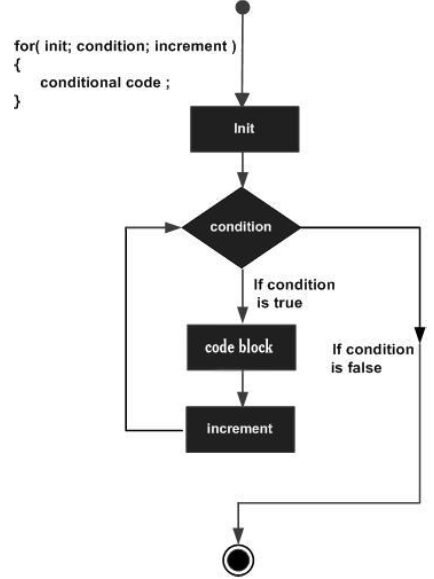# TypeScript - For Loop

The for loop executes the code block for a specified number of times. It can be used to iterate over a fixed set of values, such as an array. The syntax of the for loop is as below −

## Syntax

```for (initial_count_value; termination-condition; step) {
//statements
}
```

The loop uses a count variable to keep track of the iterations. The loop initializes the iteration by setting the value of count to its initial value. It executes the code block, each time the value of count satisfies the termination_condtion. The step changes the value of count after every iteration.

## Flowchart## Example: for loop

```var num:number = 5;
var i:number;
var factorial = 1;

for(i = num;i>=1;i--) {
factorial *= i;
}
console.log(factorial)
```

The program calculates the factorial of the number 5 and displays the same. The for loop generates the sequence of numbers from 5 to 1, calculating the product of the numbers in every iteration.

On compiling, it will generate following JavaScript code.

```//Generated by typescript 1.8.10
var num = 5;
var factorial = 1;
while (num >= 1) {
factorial = factorial * num;
num--;
}
console.log("The factorial  is " + factorial);
```

The code produces the following output −

```120
```

## The for...in loop

Another variation of the for loop is the for... in loop. The for… in loop can be used to iterate over a set of values as in the case of an array or a tuple. The syntax for the same is given below −

The for...in loop is used to iterate through a list or collection of values. The data type of val here should be string or any. The syntax of the for..in loop is as given below −

### Syntax

```for (var val in list) {
//statements
}
```

Let’s take a look at the following example −

### Example

```var j:any;
var n:any = "a b c"

for(j in n) {
console.log(n[j])
}
```

On compiling, it will generate the following JavaScript code −

```//Generated by typescript 1.8.10
var j;
var n = "a b c";

for (j in n) {
console.log(n[j]);
}
```

It will produce the following output −

```a
b
c
```
typescript_loops.htm